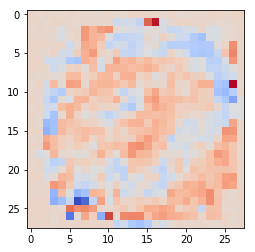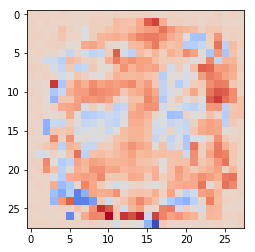In :
from torch.autograd import Variable
import torch
import numpy as np
import matplotlib.pyplot as plt

from scipy.io import loadmat

%matplotlib inline

M = loadmat("mnist_all.mat")

def get_test(M):
batch_xs = np.zeros((0, 28*28))
batch_y_s = np.zeros( (0, 10))

test_k =  ["test"+str(i) for i in range(10)]
for k in range(10):
batch_xs = np.vstack((batch_xs, ((np.array(M[test_k[k]])[:])/255.)  ))
one_hot = np.zeros(10)
one_hot[k] = 1
batch_y_s = np.vstack((batch_y_s,   np.tile(one_hot, (len(M[test_k[k]]), 1))   ))
return batch_xs, batch_y_s

def get_train(M):
batch_xs = np.zeros((0, 28*28))
batch_y_s = np.zeros( (0, 10))

train_k =  ["train"+str(i) for i in range(10)]
for k in range(10):
batch_xs = np.vstack((batch_xs, ((np.array(M[train_k[k]])[:])/255.)  ))
one_hot = np.zeros(10)
one_hot[k] = 1
batch_y_s = np.vstack((batch_y_s,   np.tile(one_hot, (len(M[train_k[k]]), 1))   ))
return batch_xs, batch_y_s

train_x, train_y = get_train(M)
test_x, test_y = get_test(M)

train_x, train_y = get_train(M)
test_x, test_y = get_test(M)

dim_x = 28*28
dim_h = 20
dim_out = 10

dtype_float = torch.FloatTensor
dtype_long = torch.LongTensor

################################################################################
#Subsample the training set for faster training

train_idx = np.random.permutation(range(train_x.shape))[:1000]
x = Variable(torch.from_numpy(train_x[train_idx]), requires_grad=False).type(dtype_float)
y_classes = Variable(torch.from_numpy(np.argmax(train_y[train_idx], 1)), requires_grad=False).type(dtype_long)
#################################################################################


x, y, and y_classes are PyTorch Variables. We will now define the neural network model. We'll use toch.nn.Sequential

In :
model = torch.nn.Sequential(
torch.nn.Linear(dim_x, dim_h),
torch.nn.ReLU(),
torch.nn.Linear(dim_h, dim_out),
)


model can be used to apply the network to Variable inputs.

We'll define a loss function using torch.nn.CrossEntropyLoss(), which computes the cross entropy from the logits of the class probability (i.e., outputs of the softmax) and the class labels (i.e., the average negative log-probability of the correct answer).

In :
loss_fn = torch.nn.CrossEntropyLoss()


### Training the model¶

We will now use Adam, a variant of Gradient Descent, to optimize the model

In :
learning_rate = 1e-2
optimizer = torch.optim.Adam(model.parameters(), lr=learning_rate)
for t in range(10000):
y_pred = model(x)
loss = loss_fn(y_pred, y_classes)

model.zero_grad()  # Zero out the previous gradient computation
loss.backward()    # Compute the gradient
optimizer.step()   # Use the gradient information to
# make a step


Now, let's make predictions for the test set

In :
x = Variable(torch.from_numpy(test_x), requires_grad=False).type(dtype_float)

In :
y_pred = model(x).data.numpy()


Let's now look at the performance:

In :
np.mean(np.argmax(y_pred, 1) == np.argmax(test_y, 1))

Out:
0.86929999999999996

Let's explore the weights a little bit. We can access the weights like this:

In :
model.weight

Out:
Parameter containing:
1.8317e-02 -8.1070e-04 -8.9239e-03  ...  -1.7976e-02 -1.6271e-02  1.3195e-02
2.3840e-02 -1.4551e-02 -7.6378e-03  ...   6.7932e-03  6.7870e-03  3.7834e-03
1.0463e-02 -1.8262e-02 -3.3359e-02  ...   7.9622e-03  1.9038e-03  3.5023e-02
...                   ⋱                   ...
1.5796e-02  7.9954e-03  1.1018e-02  ...  -2.4500e-03  6.5649e-03  2.4900e-02
3.0337e-02  2.4651e-02 -2.7405e-02  ...  -3.7025e-03 -3.4223e-02  2.2323e-02
1.2682e-02  5.7500e-03 -1.0330e-02  ...  -6.1994e-03  3.1073e-02  3.0751e-02
[torch.FloatTensor of size 20x784]

Let's look at the weights associated with unit 10

In :
model.weight.data.numpy()[10, :].shape

Out:
(784,)
In :
plt.imshow(model.weight.data.numpy()[10, :].reshape((28, 28)), cmap=plt.cm.coolwarm)

Out:
<matplotlib.image.AxesImage at 0x7fabe00f8a50>In :
plt.imshow(model.weight.data.numpy()[12, :].reshape((28, 28)), cmap=plt.cm.coolwarm)

Out:
<matplotlib.image.AxesImage at 0x7fab816d8b50>In [ ]: